# Printable Number Line 1-20Updated on Apr 12, 2021By Printablee Team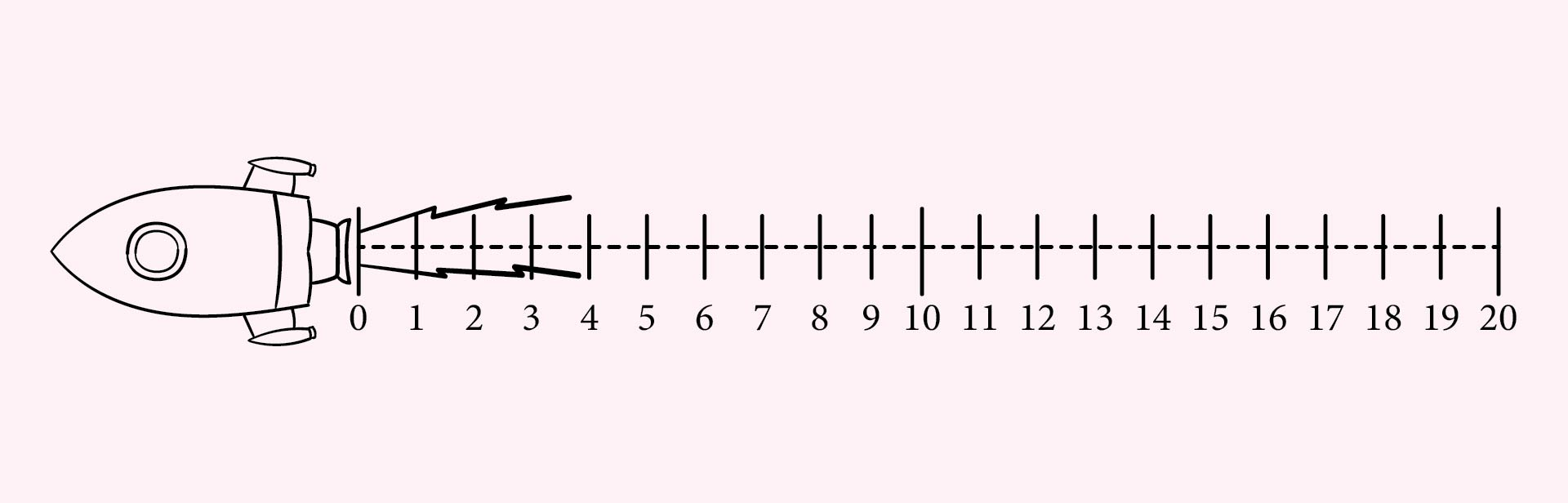## What are Natural Numbers?

The natural number is a set of numbers that are symbolized by N and are always used for counting. These numbers are also known as counting numbers because these numbers are related to mathematical calculations. These numbers have no negative numbers at all. Natural numbers are also often written on the number line.

Natural numbers are integers so natural numbers do not have decimal and fractional forms. It should also be noted that zero is not included in these natural numbers. So, natural numbers start from 1 to infinity.

## What are the Properties of Natural Numbers?

The property of natural numbers has four types: commutative property, closure property, distributive property, and associative property. Actually, those four properties make the natural numbers used in mathematics. So, let's discuss the four types of birth numbers here.

1. ### Commutative Property

The commutative of natural numbers is the result of the multiplication of two natural numbers that will remain the same even if all the sequences of the natural numbers are exchanged. An example is as follows.

• Multiplication of natural numbers: a x d = d x a
• Addition of natural numbers: a + d + d + a

Meanwhile, this commutative property cannot be used in the division and subtraction of natural numbers.

2. ### Closure Property

The next property is the closure property which shows that the multiplication and addition of several natural numbers will also produce natural numbers. Examples are 1 + 6 = 7 and 3 x 5 = 15.

Just like the commutative property above, the closure property also does not apply to subtraction and division operations. So, from the two properties above, all natural numbers only have commutative properties and closure properties for addition and multiplication only.

3. ### Distributive Property

In this distributive property, three types of natural numbers will be distributed in other calculation operations. We provide an easy example below.

If natural numbers are symbolized by x, y, z and written in the form x (y + z), the calculation of natural numbers can be done by x(y + z) = xy + xz or x(y - z) = xy - xz.

So, it can be interpreted that x is distributed on y and z. In this distributive property, natural numbers can be used in multiplication operations for addition and subtraction only.

4. ### Associative Property

The last is the associative property which explains that the addition or multiplication of any three types of natural numbers will produce the same result even if the grouping of the numbers is changed. We provide examples in the arithmetic calculation operations below.

• Addition: x + ( y + z ) = ( x + y ) + z
• Multiplication: a × ( c × d ) = ( a × c ) × d

However, this property does not apply to subtracting and dividing natural numbers.

## Natural Numbers vs Whole Numbers: What are the Differences?

Integers are numbers that start from zero and natural numbers start from 1. So, the main difference is 0. If natural numbers are symbolized as N and whole numbers are symbolized as W, the two types of numbers have these set of numbers:

• N: (1, 2, 3, 4, 5, 6, 7, and so on)
• W: (0, 1, 2, 3, 4, 5, 6, 7, and so on)

The properties of the two types of numbers are also different. Natural numbers have closure properties for multiplication and addition, but whole numbers have property closures for addition and multiplication operations.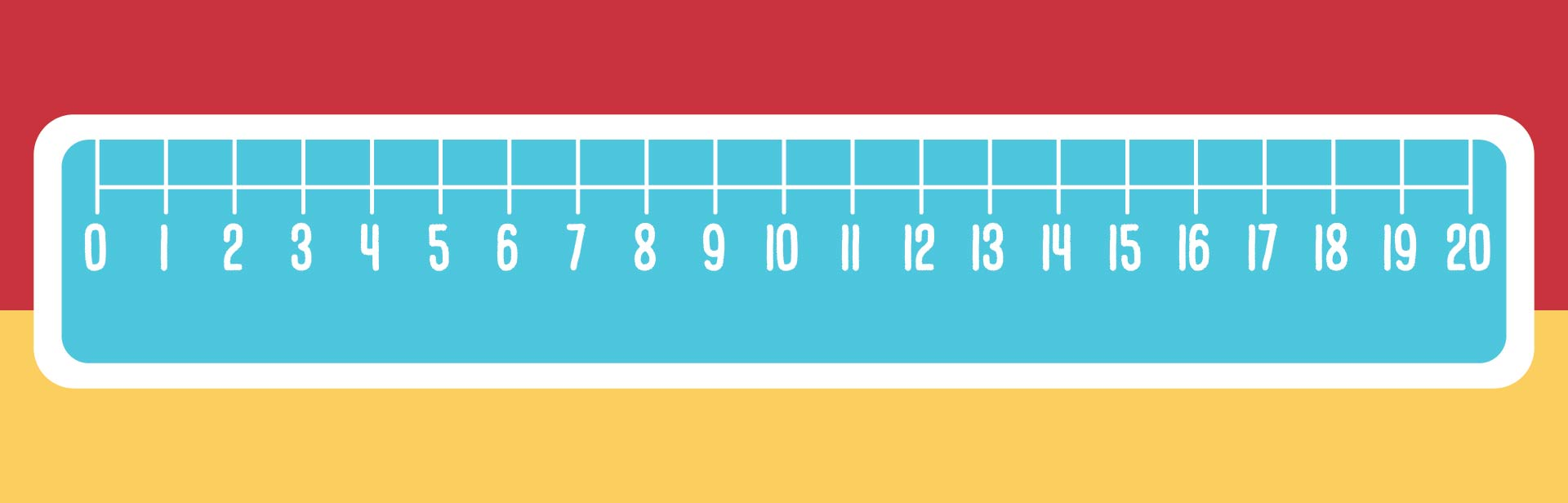We also have more printable number you may like:
12 Printable Number Worksheets
Large Printable Numbers 0-9
Printable Number Line To 50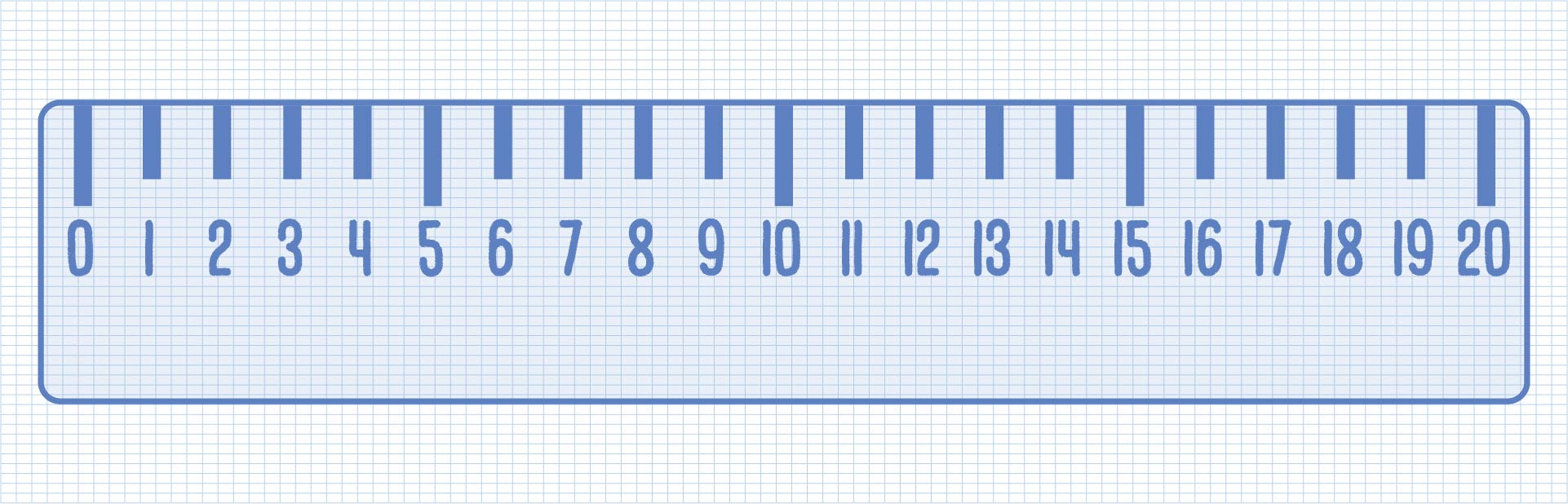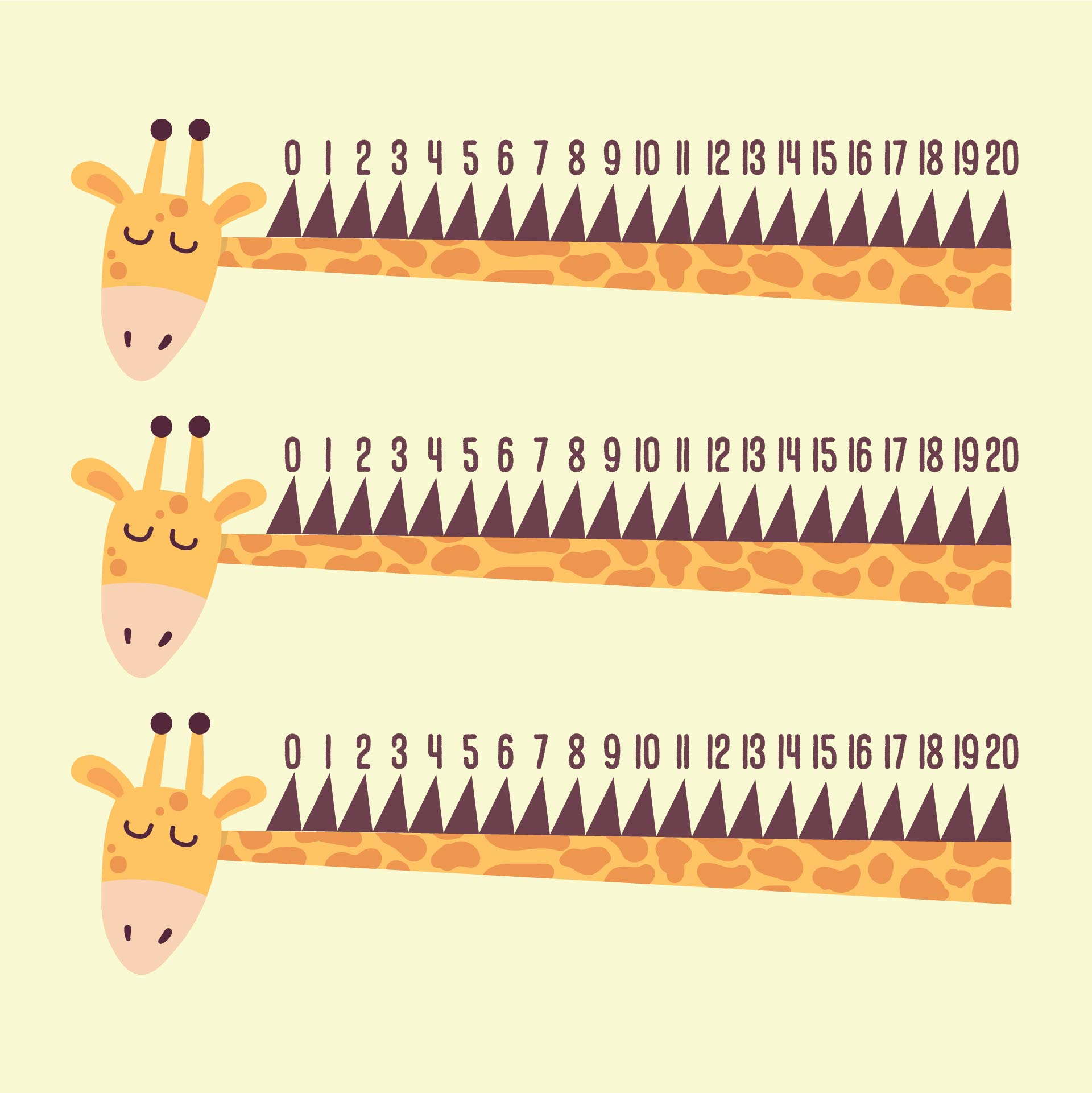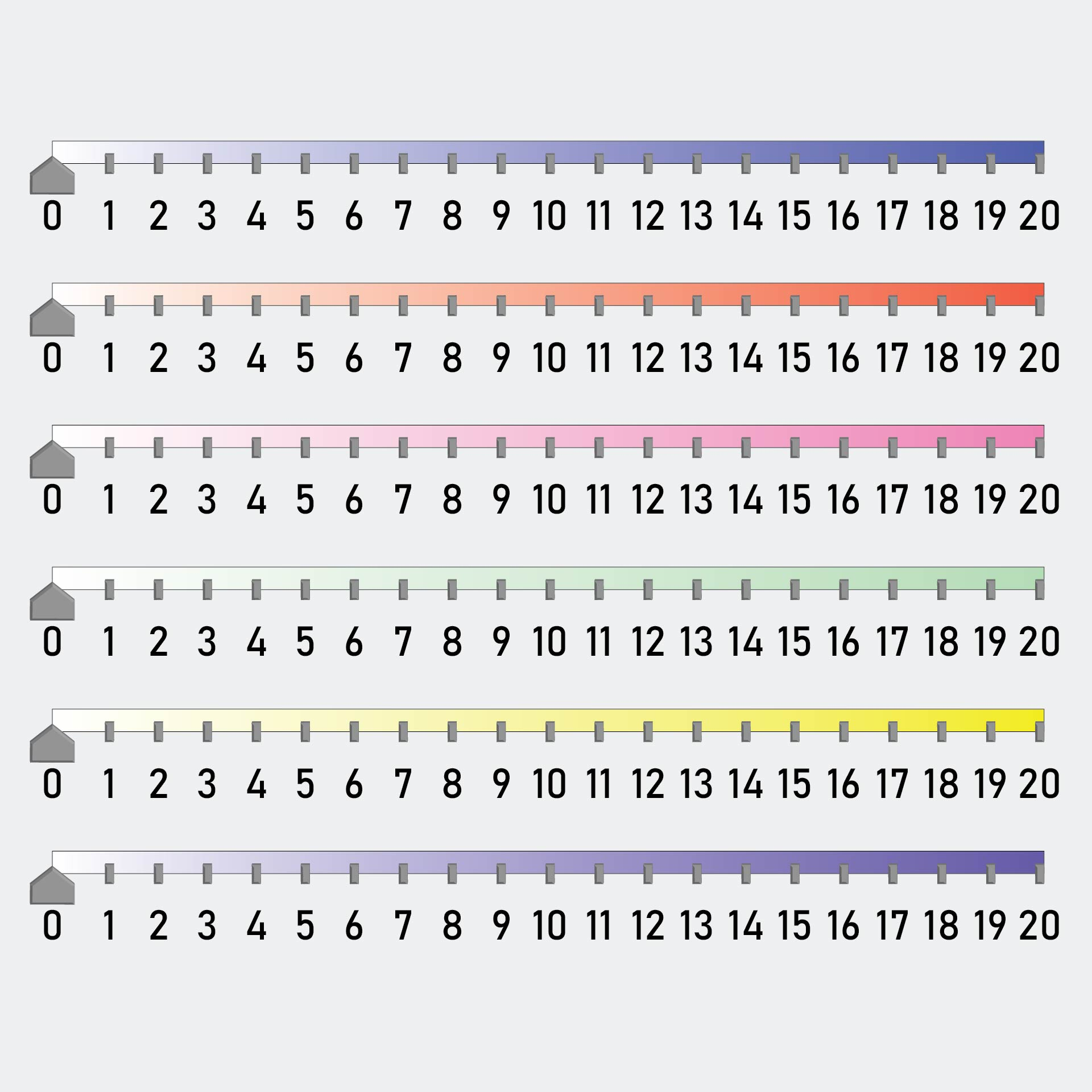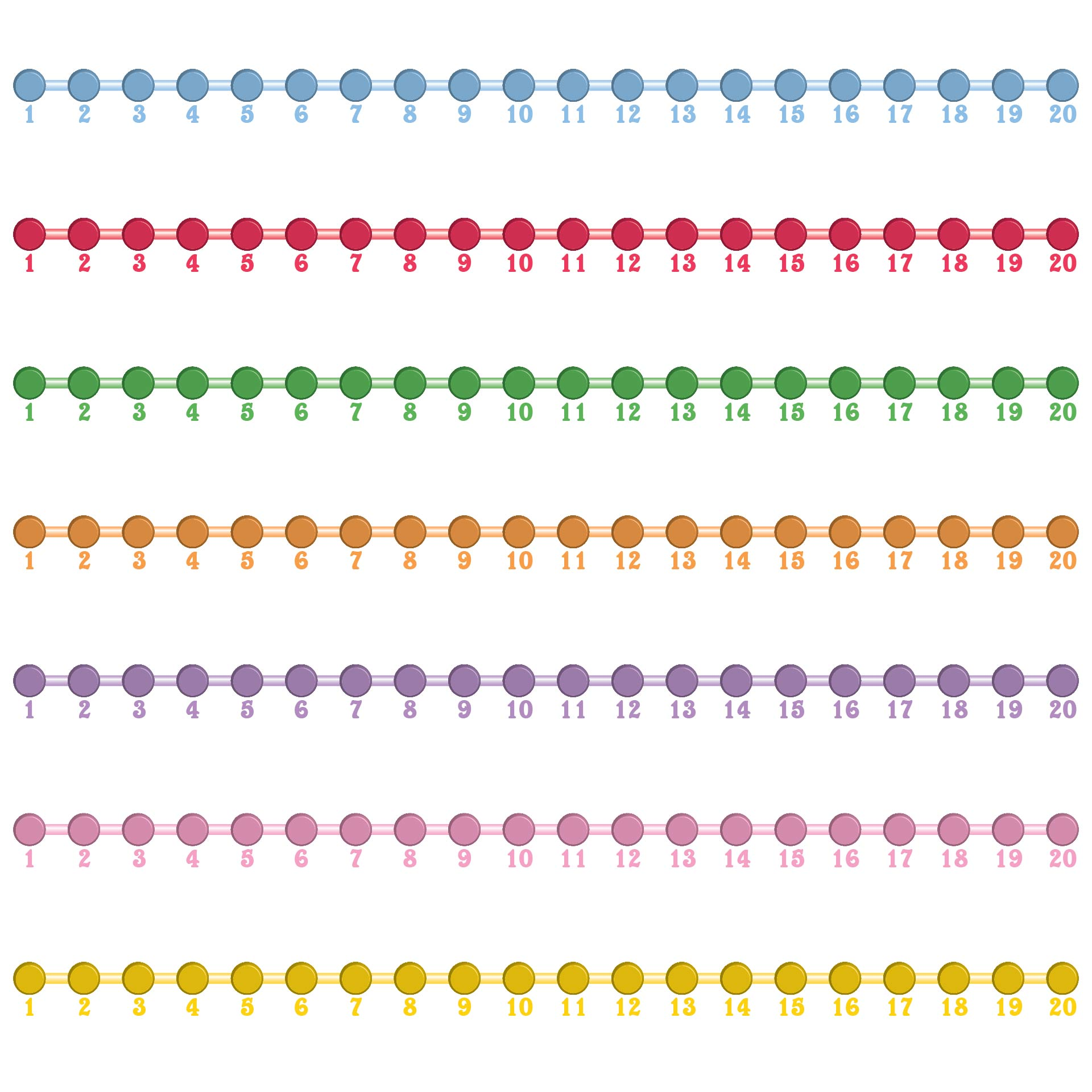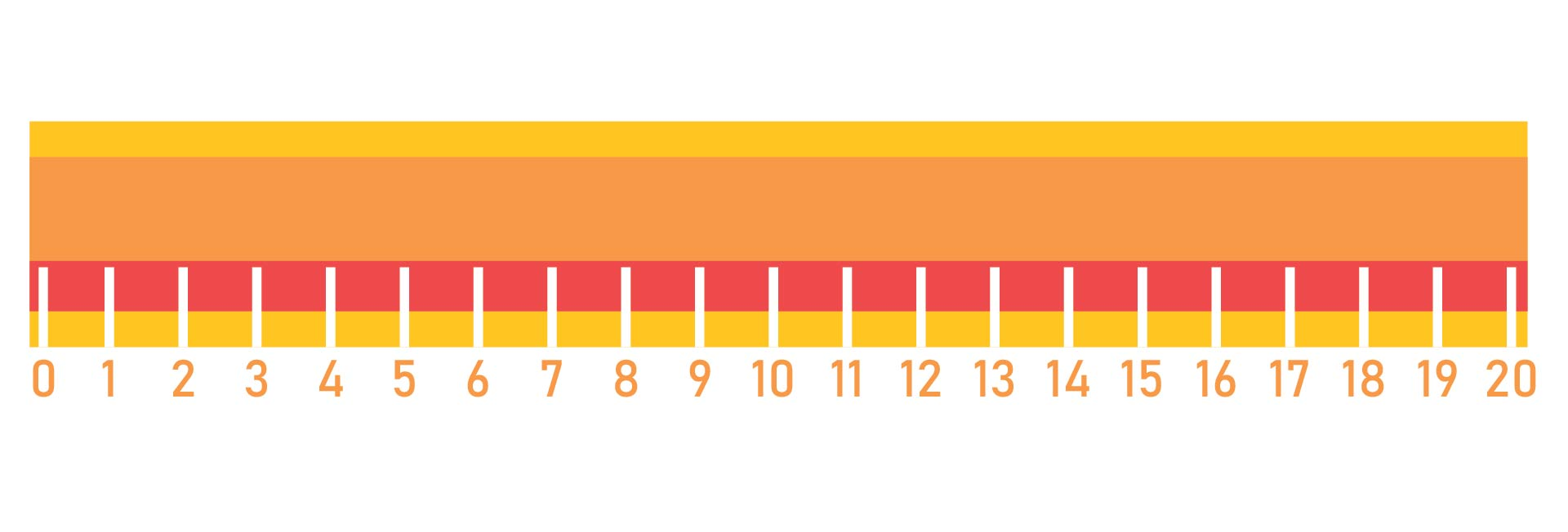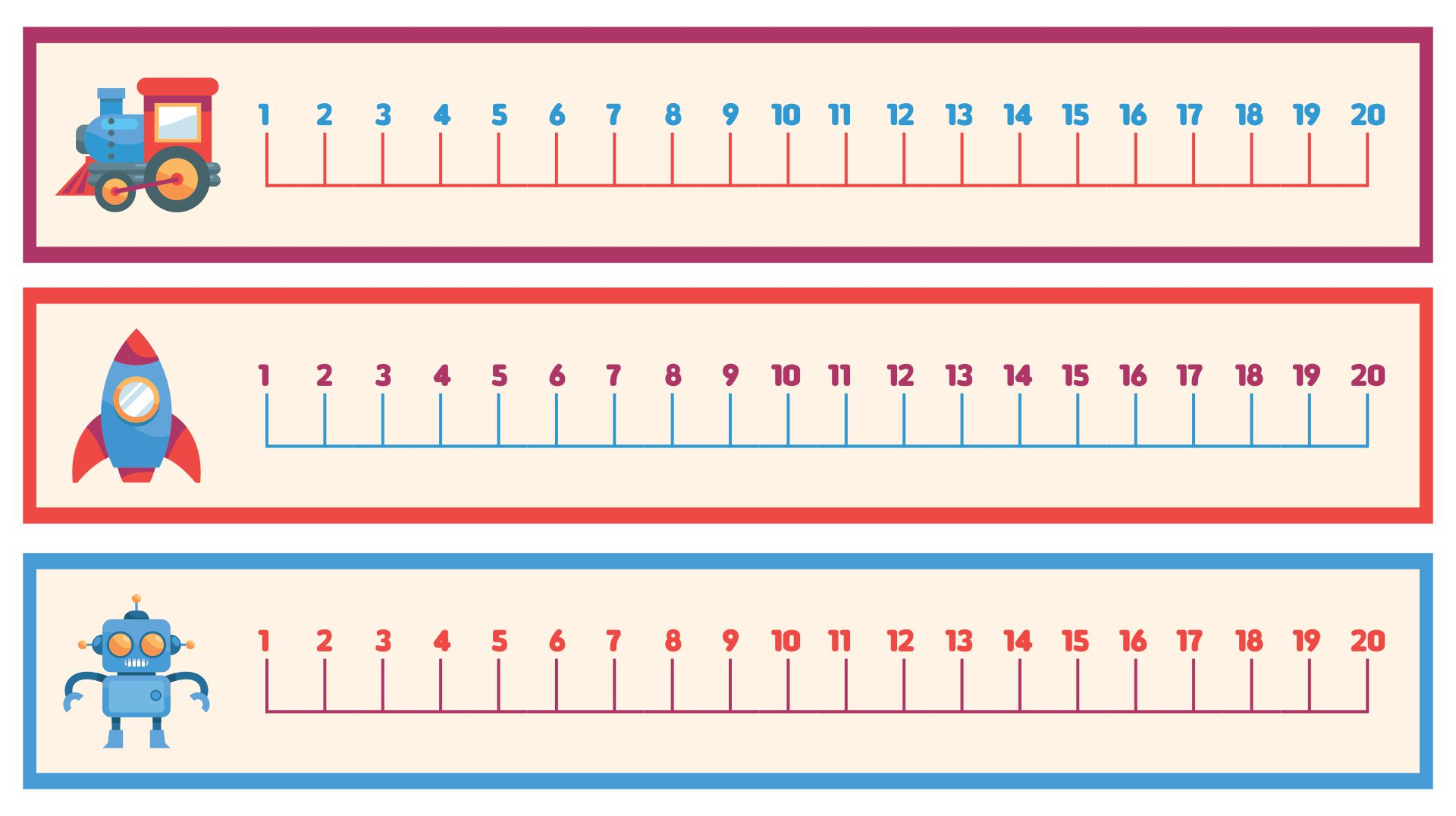## How to Teach Kids about Natural Numbers at Home?

Natural numbers are significant to learn because they are widely used in mathematical calculation operations. Therefore, you must teach children about these natural numbers. However, teaching children about natural numbers is not easy. Therefore, here we provide some easy ways to introduce and teach children about these natural numbers.

• First, teach children about the numbers 1, 2, 3, and so on. Not only teach them how to write all these numbers.
• You can use the dot method to teach them about the number. An example is the number 1 which is represented by one dot, the number 2 which is represented by 2 drops, and so on.
• You can use objects around you to explain the numbers easily. An example is using a cube-shaped box to indicate the number of each point on the box's corners. So, the total of the corners shows natural numbers.
• If they already understand it, you can explain the meaning of natural numbers to them. Use the simple explanation that we have explained above to help children understand the concept of natural numbers easily.
• After they understand natural numbers, now you can introduce the four properties of natural numbers as we have explained above. However, you have to teach them slowly.

If you want it easier, you can use a natural numbers worksheet. Natural numbers worksheets are made for children of a certain age. So, children will be able to understand the concept of natural numbers and their calculations more easily. You can also ask the children to do other natural numbers activities.

• Chalk Natural Numbers: You can write some natural numbers on the floor using chalk. Then, ask the children to write other natural numbers over the numbers you have written.
• Painting Natural Numbers: You can prepare canvas and watercolors. Then, ask the children to paint the natural numbers on the canvas with different colors.
• Number Lines: Prepare a template of the number line, whether it is blank or using some templates such as 1-20 or 1-50. Left some space blank then let children write the blank space to ensure they understand the number orders concept.